# IB DP Physics: HL复习笔记9.5.1 The Doppler Effect

### The Doppler Effect

• When a source of sound, such as the whistle of a train or the siren of an ambulance, moves away from an observer:
• It appears to decrease in frequency, i.e. it sounds lower in pitch
• Although, the source of the sound remains at a constant frequency
• This frequency change due to the relative motion between a source of sound or light and an observer is known as the Doppler effect (or Doppler shift)
• When the observer and the source of sound (e.g. ambulance siren) are both stationary:
• The waves appear to remain at the same frequency for both the observer and the source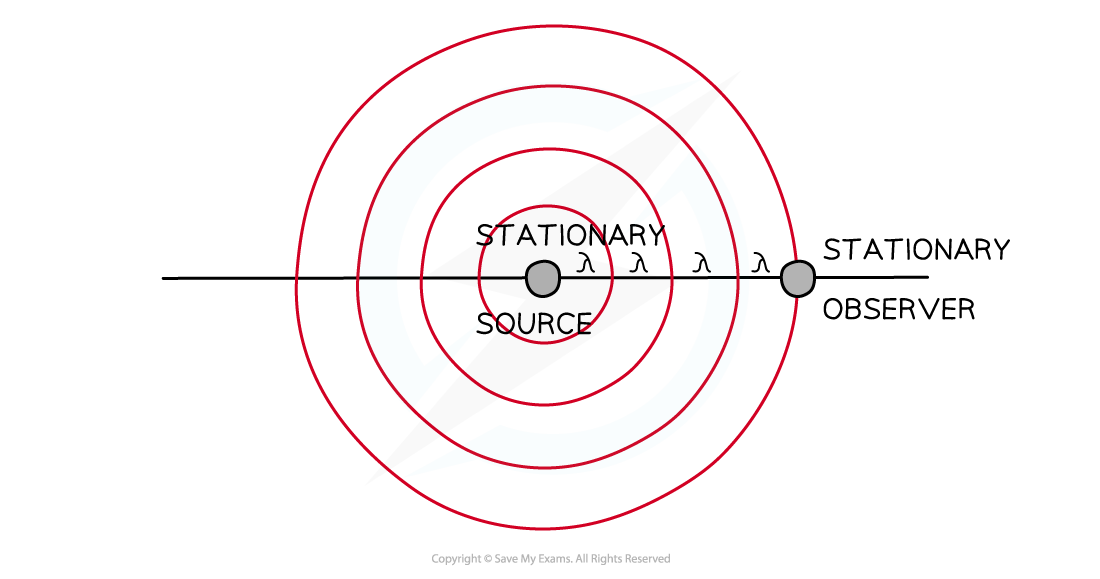Stationary source and observer

• When the source starts to move towards the observer, the wavelength of the waves is shortened
• The sound, therefore, appears at a higher frequency to the observer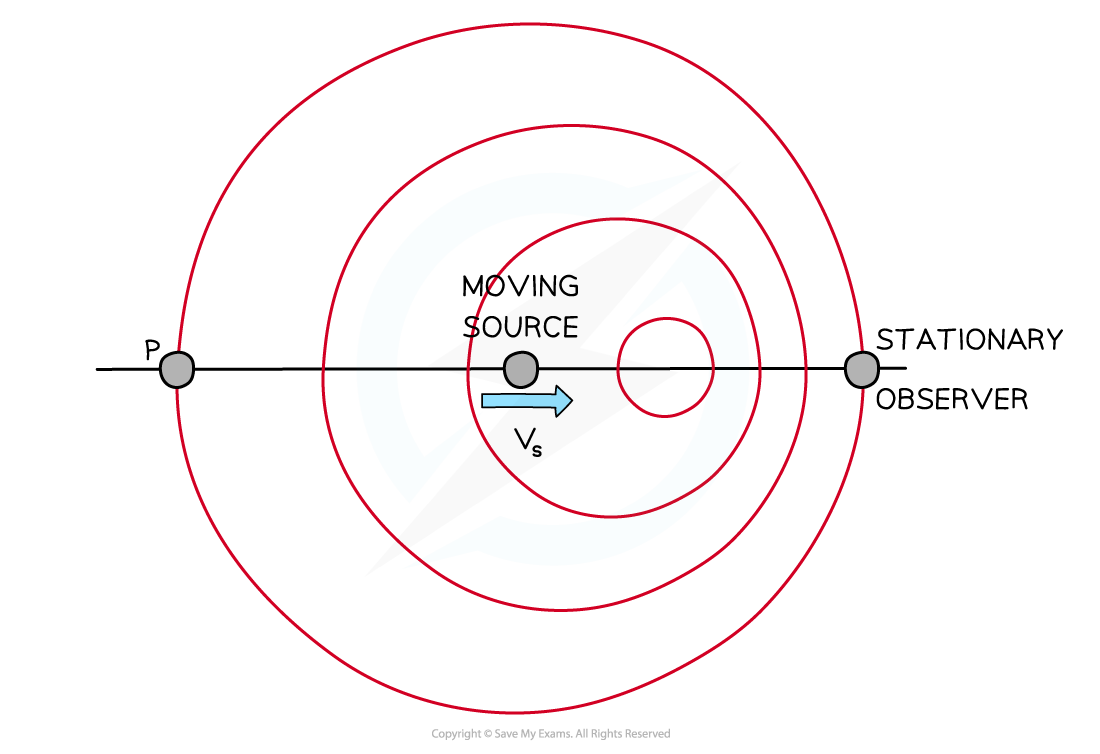Moving source and stationary observer

• Notice how the waves are closer together between the source and the observer compared to point P and the source
• This also works if the source is moving away from the observer
• If the observer was at point P instead, they would hear the sound at a lower frequency due to the wavelength of the waves broadening
• The frequency is increased when the source is moving towards the observer
• The frequency is decreased when the source is moving away from the observer

#### Worked Example

A cyclist rides a bike ringing their bell past a stationary observer. Which of the following accurately describes the doppler shift caused by the sound of the bell?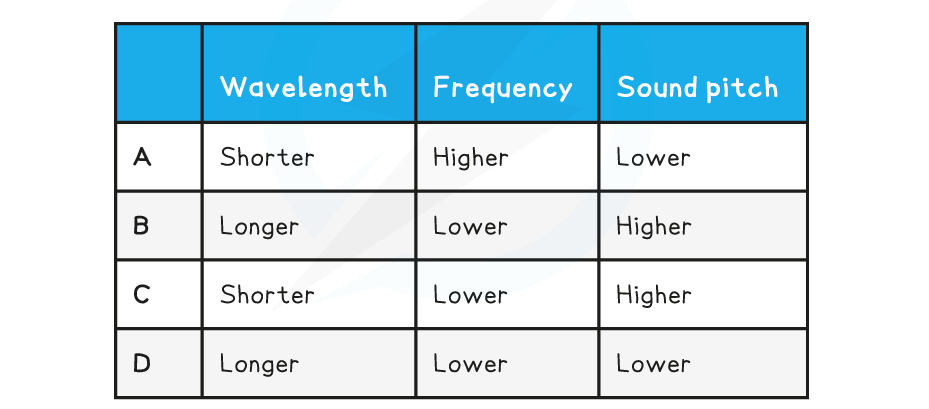• If the cyclist is riding past the observer, the wavelength of sound waves are going to become longer
• This rules out options A and C
• A longer wavelength means a lower frequency (from the wave equation)
• Lower frequency creates a lower sound pitch
• Therefore, the answer is row D

### Representing The Doppler Effect

• Wavefront diagrams help visualize the Doppler effect for moving wave sources and stationary observers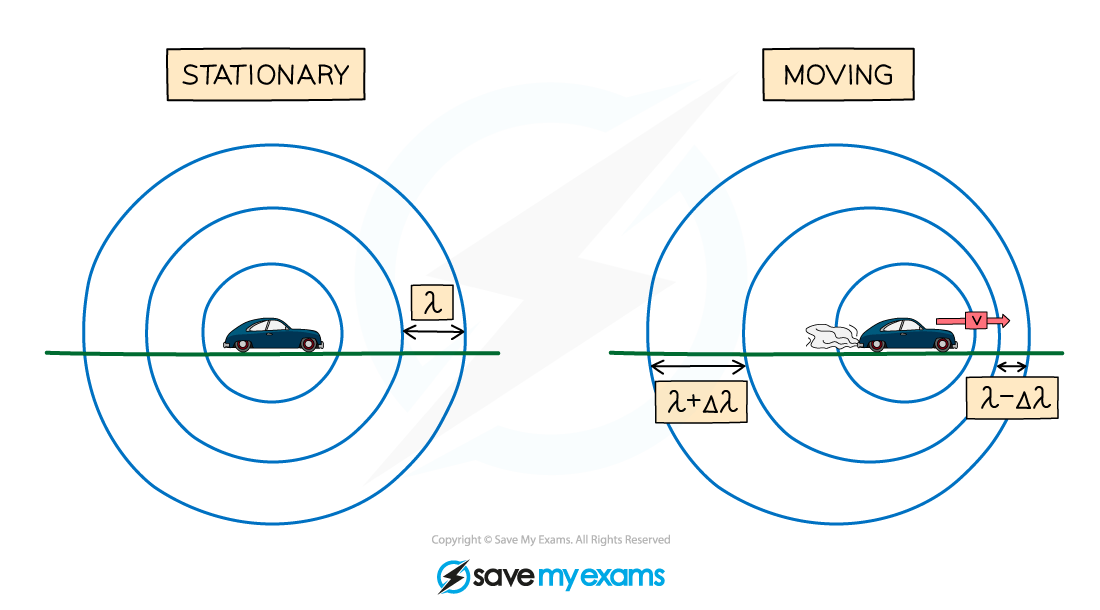Wavefronts are even in a stationary object but are squashed in the direction of the moving wave source

• A moving object will cause the wavelength, λ, (and frequency) of the waves to change:
• The wavelength of the waves in front of the source decreases (λ – Δλ) and the frequency increases
• The wavelength behind the source increases (λ + Δλ) and the frequency decreases
• This effect is known as the Doppler effect or Doppler shift
• Note: Δλ means 'change in wavelength'
• The Doppler shift is observed by all waves including sound and light
• When the source starts to move towards the observer, the wavelength of the waves is shortened
• For sound waves, sound, therefore, appears at a higher frequency to the observer
• For light waves, the light shifts towards blue due to its higher frequency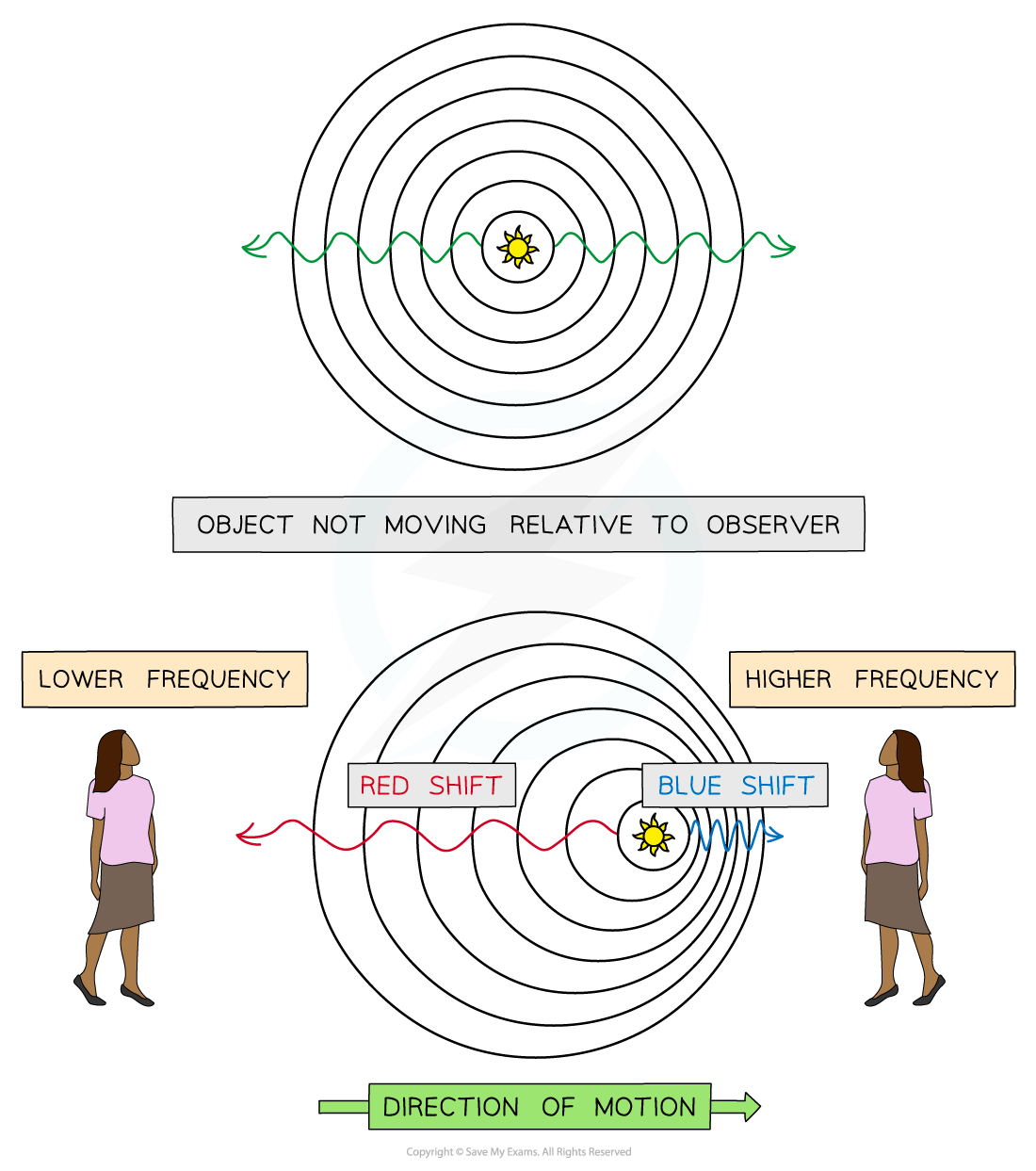Representing red-shifted and blue-shifted light

• When the source starts to move away from the observer, the wavelength of the wave broadens
• For sound waves, sound therefore appears at a lower frequency to the observer
• For light waves, the light shifts towards red due to its lower frequency
• When the source starts to move towards the observer, the wavelength of the wave shortens
• For sound waves, sound therefore appears at a higher frequency to the observer
• For light waves, the light shifts towards blue due to its higher frequency
• This is because red light has a longer wavelength than blue light